﻿ Model Selection for Count Data with Excess Number of Zero CountsPublications are Open
Access in this journal
Article Versions
Export Article
• Normal Style
• MLA Style
• APA Style
• Chicago Style
Research Article
Open Access Peer-reviewed

### Model Selection for Count Data with Excess Number of Zero Counts

K.M. Sakthivel , C.S. Rajitha
American Journal of Applied Mathematics and Statistics. 2019, 7(1), 43-51. DOI: 10.12691/ajams-7-1-7
Received November 17, 2018; Revised December 28, 2018; Accepted January 15, 2019

### Abstract

Zero inflated models have been widely studied in statistical literature. Zero inflated Poisson model and hurdle model are the most commonly used models for modeling the overdispersed count data. In adddition to this, recent studies shows that a nonparametric and data dependent technique known as artificial neural networks (ANN) produce better performance for modeling the over dispersed and zero inflated count data. In this paper, we compared the performance of different models such as zero inflated Poisson model, hurdle model and ANN for modelling the zero inflated count data in terms of standardized MSE, SE, bias and relative efficiency. An application study is carried out for both the simulated data set and real data set. Also for checking the suitability of these three models, we verified the group membership of the models, by adopting three classification techniques known as discriminant analysis, CART and random forest. We proposed an algorithm for selecting the better model among a set of models and computed the misclassification rates for a zero inflated count data set using different classifiers.

### 1. Introduction

A critical question faced by data analysts while modeling the count data is how to choose a suitable model for a particular study. For modeling the categorical count data with excess zero counts, numerous choices of methodologies have been used by various researchers in literature. Usually Regerssion models are widely applied for modeling this kind of data. However other data analyasis techniques also has been adopted in the recent years, which includes machine learning techniques like artificial neural networks (ANN), CART etc. However the major problem encountered is the selection of most suitable model for analysing the count data, since various methods provides dissmimilar results, which also varies from one data to another data. One of the widely accepted and used methods for modeling the categorical count data with excess zero counts is the zero inflated regression models, which supply a broad and rigorous area of reasearch 1. In order to properly describe the characteristic of excess of zeros in the count data, zero inflated models are considered to be more convenient compared to the standard regression models. The concept of zero inflation was first commenced by Neyman 2 and Feller 3. The zero inflated version of a Poisson regresion model was presented by Lambert 4 as a more pragmatic way for handling the count data with large amounts of zero counts. Yip and Yao 5 provided several parametric zero inflated count distributions for accomodating the surplus zero counts in the insurance claim data. A zero inflated generalized Poisson regression model was introduced by Famoye and Singh 6 for analysing a domestic violence data with excess number of zeros. Hurdle models 7 and two part models 8 are some of the other models strongly associated with zero inflated models. Also recent studies shows that a nonparametric and data dependent technique called ANN can be used for count modeling, 9. For model comparison using standardized MSE, bias and SE, we adopted a simulation study as well as a data study using an existing zero inflated count data set. Also we utilized diagramatic representation of standardized MSE values of different models for depicting the efficiency of models for analysing the zero-inflated count data.

But the appropriate model selection plays a significant role in count data modeling. Most of the studies use mean squared error (MSE), bias, standard error (SE) and root mean square (RMSE) etc for comparing different count data modeling approaches and summarize the result based on these values. One of the most critical problem encountered while adopting this measures for model comparison is that sometimes various models produce different outcomes while changing the data. Hence a model selection criteria is inevitable for the practisioners for finding a most suitable approach for modeling the zero inflated count data. Usually in statistical literature discriminant analysis is used for classification of models in to any one of the various possible classes and thereby misclassification rates can be calculated and taking in to account this misclassification rates, we can determine a most appropriate model among several possible models 10. Later machine learning algorithms are also adopted for classification purpose. ANN is one among them and it takes considerably large amount of learning time and the network is trained based on the selection of parameters like the convergence rate, number of hidden layers etc. Furthermore classification and regrssion trees (CART) are also used as classifiers which provides interpretation very easily and rapidly while comparing to ANN, but its disadvantages are lower performance for high-dimensional data and shows tendency to overfit the training data 11, 12. Random Forest classifiers are another classifier which use an ensemble of number of CART and has several advantages over other classifiers 13. So that in our paper we also proposed an algorithm to find an appropriate model among a set of models for modeling the count data with excess zero counts by classifying the mean squared values of different models using discriminant function analysis, CART and random forest.

Organisation of the paper is as follows. Section 2 provides a brief description about various count models for modeling the count data with excess zeros. A simulation study and a data study is performed in section 3 for comparing various count models in terms of standardized MSE, SE and bias. In section 4 describes about different classifiers for selecting the appropriate model and provides a model selection criteria for selecting the best model among a set of models for count data modeling with the help of various classifiers like discriminant function analysis, CART and random forest. Section 5 concludes the results of the study.

### 2. Zero Inflated Count Models

This section provides a brief discription about conventional parametric models for modeling the excess zero counts such as zero inflated Poisson regression model and hurdle Poisson regerssion model and a nonparametric method called artificial neural networks for zero inflated count data modeling.

2.1. Conventional Zero Inflated Models

Zero inflated models are latent class models proposed for handling the data which shows two kinds of zeros. It is basically a two part model with specific behavioral interpretation. These models are widely accepted by various experts in the domain of count modeling with excess of zero counts. For any zero inflated count model the PMF can be written in the form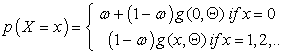Here the variablerepresents the count random variable anddenotes the probability mass function of the variable. The zero inflation parameter is represented by the notation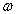and it always lies between zero and one. Zero inflated distributions mainly focussed on handling the overdispersed count data with many zeros. Mullahi 7 first discussed a two part model for handling the count data with excess number of zeros. Another work related to zero inflated models was zero inflated Poisson (ZIP) model by Lambert 4.

2.1.1. ZeroInflated Poisson (ZIP) Regression Model

In order to handle the zero inflated count data, Lambert 4 introduced a mixture distribution by combining a degenerate at zero distribution and a Poisson distribution. This distribution is suitable for handling the count data with purely overdispersion and zero inflation features. Lambert 4 provided the specification of the ZIP distribution as followsHere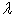represents the mean of the Poisson distribution which is greater than zero anddenotes the zero inflation parameter which is always lies between zero and one. ZIP distribution have two components, one component is for admitting excess zero counts ratioand the proportion of zeros coming from Poisson distribution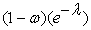and the second component admits positive counts generated from the zero-truncated Poisson distribution. In ZIP regression model two distinct processes are used for estimating the proportion of zero countsand the mean parameter. For classifying the structural zeros from other zeros a logit model is used and a log-linear model is applied for modeling the counts from a Poisson process. Usually in this model a canonical link for the Poisson model is considered for the parameter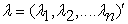which satisfy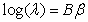and the parameter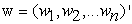has the canonical logic link function as follows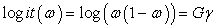where B and G are the covariate matrices. For parameter estimation of Poisson regression model, maximum likelihood approach is used. Since closed form solutions do not exist for the partial derivative equations, Newton-Raphson algorithm or EM algorithm can be used for estimating the parameters of the model.

2.1.2. Hurdle Poisson Regression Model

This is another widely accepted model for modeling count data with excess zero counts. This model admits all zero counts in one part and all positive counts at another part of the model. So that this model can be considered as a superior model, since this model handles zero counts and non-zero counts separately. This model utilizes binomial practice by recognizing either the count random variable attain the value zero or positive value. Usually the second part admits positive counts from a zero truncated Poisson or negative binomial distribution. In this paper, we considered Poisson hurdle specification for modeling the count random variable. It can be written in the form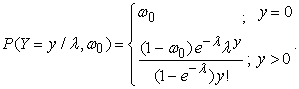The hurdle is crossed if the count variable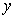shows the value greater than zero and for handling the positive values a zero-truncated count model is used. The probability of hurdle clearance for generating non zero counts are denoted byThis model considers a complimantary log-log link function for the proportion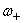and a log link function for the parameteras follows.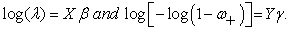This model returns a standard Poisson model if the the values of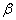and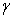are equal.

2.2. Artificial Neural Networks

Artificial neural networks has been used in the field of count modeling by various researchers 9, 14. One of the most popular architecture of ANN is multilayer perceptron (MLP). Usually in MLP, back propagation (BP) algorithm is used for learning process by minimizing the sum of squared errors. Due to the generality of ANN, this model produces precise and accurate prediction in almost all situations which is inevitable in most of the applications such as insurance, medicine, epidemiology etc. According to Young II et al. 15, ANN can be able to show the complex input and output non-linear associations. In order to build ANN, the number of nodes or neurons, a method for relating the neurons and a learning algorithm must be fixed. Usually ANN model is represented as a combination of three or more layers, which interconnects the processing elements called neurons. The first layer contains the input observations; last layer is the output layer which produces the output. In between there are one or two layers called hidden layers which are used for learning and tracing the complex patterns regulating the network’s data. And for controlling the signals passing through the network, an activation function is applied. Using a training sample the weights of the network has been initialized and these weights are usually used for prediction of the training sample. The neurons or artificial neurons represent a device with one output and many inputs. Usually ANN produces an outputby adopting a set of input observations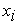with the help of a specified number of hidden layers. The architecture of an ANN model with a single hidden layer can be written as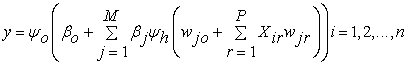where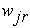represents the weight for the input connectionat the hidden node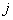.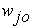is the bias for the hidden node and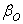is the bias for the output nodes.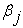also represents the weight dependent to the hidden nodeThe number of covariates and the number of nodes in the hidden layer are represented by the symbolsandThe functionsand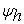denotes the activation functions of output layer and the hidden layer respectively.

### 3. Model Performance Analysis of ZIP, Hurdle and ANN

In this chapter, we considered ZIP regression model, hurdle Poisson regression model and ANN for modeling categorical count data to evaluate the performance of the models using the measures standardized MSE, SE and bias. We conducted two experiments for comparing the performances of these models. For this purpose, we considered a data set from the package Insurance Data from R software. In the first experiment, we conducted a simulation study using ZIP distribution for generating random samples. This set of generated values and secondary data in our hand, we formulated simulated panel data set. In the second experiment, we conducted a data study using car insurance data set available in R for evaluate the performance of the above mentioned models. We plotted the values of standardized MSE, SE and bias of different models with respective to inflation rate to analyze the efficiency of models under study.

3.1. Experiment 1: Simulation Study

We conducted a simulation study to compare the performance of ZIP, hurdle and ANN models for modeling zero inflated count data in terms of standardized MSE, SE and bias. We used the ZIP model for generation of counts for a given value of parameters and randomly pick those counts from our secondary data in order to get the categorical data. The simulation study is conducted using the following steps.

1. Generate a random sample from ZIP forandwhere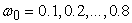.

2. Generaterandom samples for each of size n = 100, 250, and 500 as discussed above.

3. Calculate standardized MSE by using actual and estimated claim counts for the models ANN, hurdle and ZIP as follows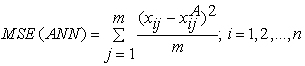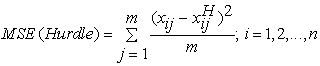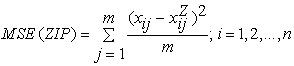where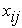represents claim numbers of the test set of observed values and,and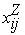represents the estimated values of claim counts for the models ANN, hurdle and ZIP respectively.

4. The relative efficiency of ANN with respect to hurdle and ZIP models are obtained as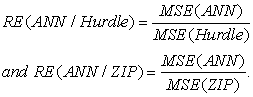The sample variances are computed as follows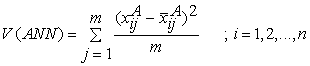5. where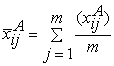,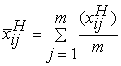and6. The average biases of the predicted values for ANN, hurdle and ZIP are evaluated as follows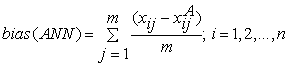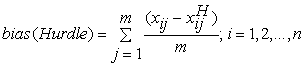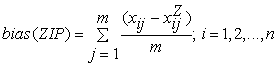7. Steps (1) to (6) are repeated for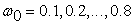andsamples.

For ANN, the number of claims is considered as target variable and other variables are considered as input variables. standardized MSE, SE and bias are obtained using two hidden layer (3,1) network for ANN. The results of simulation study for sample size (i.e., n = 100) for 50 replication (i.e., m = 50) are given in the following Table 1.

The relative efficiency of ANN over hurdle and ZIP provided in Table 1 shows ANN performs relatively better than ZIP and hurdle models. From Figure 1, Figure 2, Figure 3, Figure 4, it is observed that the values of standardized MSE, SE and bias of ANN is consistently decreasing and also minimum compared to hurdle and ZIP models for higher values of the inflation parameter. Hence it is concluded that ANN performs relatively better than hurdle and ZIP except= 0.1. Further, we conclude from the relative efficiencies that ANN provides better fit compared to hurdle and ZIP for modeling zero inflated and over dispersed count data.

• Figure 1. Standardized MSE of ANN, Hurdle and ZIP for simulated data set (n=100)
• Figure 2. SE of ANN, Hurdle and ZIP for simulated data set (n = 100)
• Figure 3. Bias of ANN, Hurdle and ZIP for simulated data set (n = 100)
• Figure 4. Relative efficiency of ANN, Hurdle and ZIP with respect to inflation parameter for simulated data set (n=100)
3.2. Experiment 2: Using Secondary Data

In this study, we considered the car insurance data set available in the package of InsuranceData in R software. The data set contains total records for a period of three years which takes account of the claim file with 1,20,000 records. Our aim is to model the number of claims which depends on three categorical variables namely driver’s age category, vehicle value and period. The frequency distribution of the number of claims is given in Table 2 and its frequency plot is provided in Figure 5. It is observed that the frequency of zero is very high compare to other counts in the data. Further, it is observed that 86% of the values are zeros and the dispersion index is 3.516. Hence the data under study is over dispersed.

• Figure 5. Frequency of claim count

The data analysis is performed using R software for different percentages (10%, 20%, 30%, 40%, 50%, 60%, 70%, 80%, 90% and 100%) of the data. We used two different ratio for training and testing as 70%: 30%, and 80%: 20% and calculated the standardized MSE and RE for ZIP, hurdle and ANN models. For ANN modeling, we used back propagation algorithm since it provides consistent and fast convergence with two hidden layer. The outcome of this experiment is given in Table 4.

From Table 4, it is observed that ANN performs better than ZIP and hurdle models for 75% (15 out of 20) of the trials. In this study, we have calculated standardized MSE for all the three models. The standardized MSE of ANN is relatively smaller compared to ZIP and hurdle models. While comparing the average relative efficiency from Table 5, ANN performs better than ZIP and hurdle models for this particular over dispersed count data. Figure 6 provides relative efficiency of model performance of ZIP, hurdle and ANN with respective inflation rate. It is observed that ANN performs better than ZIP for moderate inflation rate and always better or as good as hurdle model. ZIP over take the hurdle model for lower inflation rate and equally performs for moderate and higher inflation rate.

• Figure 6. RE of ANN, Hurdle and ZIP

### 4. Model Selection for Count Data using Classification Techniques

In this information era, the advent of new technology for the storage and retrievel of voluminous of data is made easy. Further the quantum of count data is also huge and with micro details. As a result, the existing traditional count models sometimes fails to analyse this big data. Hence the processing and analysing of the big data is new and big chanllenges for the statistician especially for model building aspect. In this section, we proposed a methodology based on classification techniques and new algorithm for the selection of appropriate and efficient model for the given set of inputs for modeling count data. In this connection, we have identified three classification methods namely discriminant analysis, classification and regression tree (CART) and random forest method by training the system to learn by feeding past data for finding a best model for the given set of inputs for modelling the count data.

Usually discriminant analysis is used for classification of models in to any one of the various possible classes and thereby misclassification rates can be calculated and taking in to account this misclassification rates, select appropriate model among several possible models 10. Classification and regrssion trees (CART) are also used as classifiers which provides interpretation very easily and rapidly while comparing to ANN, but its disadvantages are lower performance for high-dimensional data and shows tendency to overfit the training data 11, 12. Random Forest classifiers are another classifier which use an ensemble of number of CART and has several advantages over other classifiers 13. The more details about these three classification techniques is given in the following sections.

4.1. Discriminant Function Analysis

It is a supervised classification technique that entails the usage of a set of certain methods, algorithms and techniques with the aim of determining those features of objects that have the maximum significance concerning the classification of objects associated with a population in to predetermined classes and to establish the classification of new objects in to classes which are predefined. Also this method aims to determine the variables with highest discriminatory power, hence it helps to determine the most appropriate variable for the classification of objects in to specific classes. The discriminant function is used for class separation, which are defined with respect to the descriptive variables of objects and used for determining the discriminant variables. The association between the three crucial elements of discriminant analysis can be summarized as follows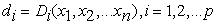whereis the discriminant variable,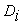is the discriminant function and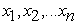are the descriptive variables. The two phases representing the process of a discriminant function are

a) Test the significance of a set of discriminant function and

b) Classification

In this method an algorithm is required for the classification of objects in to specified classes. Basically there are two types of discriminant analysis

a) Linear discriminant analysis (LDA) and

Linear discriminant analysis method was proposed by Fisher 10 as a method for classifying the objects or observations in to one of the two specified groups which is usually mutually exclusive and exhaustive in nature.

And this classification is based on a linear function called discriminant function which is based on a set of independent variables related with each object. This linear function is preferred to exploit the group separation metric. The important variables which help to classify the given observations in to any of the several groups might be identified while computing this linear function and then this discriminant function can be used to classify the new observations in to any of the predefined groups. The assumption underlying LDA are that for all classes the covariance between the independent variables is equal. While quadratic discriminant analysis does not satisfy the equal covariance assumption across classes. Usually we use the training set which is a randomly selected portion of the data to build the model and the remaining portion called testing set is used for evaluating the accuracy of the model.

4.2. CART

This methodology is introduced by Breiman et.al, 16 and is technically recognized as binary recursive partitioning. CART modeling process divides the data set in to two exact subgroups that are more identical with respect to the response variable than the initial data set, hence this model is considered as binary. It is recursive because each of the resulting subgroups or nodes, the process is repeated. The resulting model is named as a decision tree or simply tree. If the data set is satisfactorily large, CART model builds a model on a particular part (randomly selected part) of the data called learning sample and then test it on the outstanding part of the data called test sample. In this mechanism, the tree building is done using the learning sample and the test sample is used to estimate the misclassification rates and to prune the tree accordingly. The predictive power of the model can be enhanced by this self testing procedure of model building. A tree diagram is usually used for representing the resulting model. It can provide very close estimates of the response to the actual responses, since it divides the data in to a set of a number of non-overlapping subgroups or nodes. Ability to deal with missing values and being unaffected by outliers are the important features of CART model.

4.3. Random Forest

It is one of the classification method from the set of most popular classification algorithms. As the name implies it is nothing but an ensemble of classification trees. Instead of growing a single tree in CART model, in this method each of the classification trees is grown using a bootstrap sample of the data and a vector of arbitrarily chosen subset of features is considered at each split 12, 13, 16. Thus random forest (RF) method uses both bootstrap aggregation or bagging and random variable selection for tree building. For obtaining low bias trees each tree is grown fully, simultaneously random variable selection and bagging provides low correlation of the individual trees. Thus the algorithm provide an ensemble that can realize both low variance and low bias by taking the average over a large ensemble of trees with low bias, high variance but low correlation. It has some enhancing advantages like relative robustness to outliers, higher classification accuracy, efficiency in handling high-dimensional small sample data and internal feature selection that makes it ideal for classification 13.

4.4. Algorithm for Model Selection for Modeling Count Data

Classifiers like discriminamt function analysis, CART and Random forest are used to identify and classify the observation into particular population among the set of populations. Here we have used some features of these classifiers to identify and select a suitable model among a set of models by considering the past outcome from various count models. For that we adopted a step by step procedure for finding the suitable model for modeling over dispersed count data using these three classifiers. The steps are given below

Step 1: Partition the data for training and testing for a particular proportion.

Step 2: Finding the mean square values between expected frequency and observed frequency for the test set using ZIP regerssion model, hurdle model and ANN

Step 3: Find the misclassification rate for all three classification methods (discriminant analysis, CART and random forest).

Step 4: Repeat step 1 & 2 for different proportion of training and testing.

Step 5: Find out the best model using the classification result.

Adopting this step by step procedure we can obtain the appropriate model for count data by utilizing the misclassification rates.

4.5. Application

Here we considered two populations for model evaluation. As the first population we randomly select 20% of the simulated car insurance data set (data set has been provided in section 3.2). The whole data set is considered as the second population. The analysis in terms of standardized MSE is performed for different percentages (20%,40%,60%,80% and 100%) of the population 1 and performed analysis for 70:30 and 60:40 ratio of partition of the data set and the standardized MSE values also obtained for different hidden layers (2,3,4) for ANN. Similarly for the population 2 computation of standardized MSE values for different models are done by considering every additional of 10% from 10% to 100% of the data using training testing ratio (80:20 and 70:30). Figure 7 and Figure 8 shows the relative efficiency of the models for population 1 and population 2.

• Figure 7. Ratio of Standardized MSE values of ANN, Hurdle and ZIP for population 1
• Figure 8. Ratio of Standardized MSE values of ANN, Hurdle and ZIP for population 2

By utilizing standardized MSE values obtained for population 1 and population 2, we attempt to find a better model using the classifiers discriminant analysis, CART and Random forest. For classifying the standardized MSE values of ANN, hurdle and ZIP in population 1, we considered sample size, training testing ratio percentage of partitioning the data and number of hidden layers in neural network as independent variables and for population 2 we considered only the sample size and training set percentage (while partitioning the data) as independent variables for finding the overall misclassification rate.

We obtained the misclassification rate of standardized MSE values of three models (ANN, Hurdle and ZIP) using three classifiers Discriminant analysis, CART and random forest. The misclassification rates of predicting the group membership of standardized MSE values of ANN, hurdle and ZIP are given in Table 6. This shows that for both populations the misclassification rate using various classifiers are negligible. Hence based on this result and figures, we can also conclude that ANN provides superior fit to the count data with excess zero counts.

### 5. Conclusion

In this study, we analyzed the performance of three popular count data models for modeling the zero inflated count data. We briefly reviewed these models and presented a simulation study for preferring a most suitable model among ANN, hurdle and ZIP models by comparing the measures standardized MSE,SE, bias and relative efficiency, while modeling the zero inflated count data when the data generated from the ZIP distribution. The results of the simulation study shows that ANN provides relatively better performance compared to hurdle and ZIP models. The study has been extended for already existing zero inflated categorical count data set and obtained the results. The outcomes shows that for this data set also ANN provides relatively better performance in terms of standardized MSE and RE. For obtaining the group membership for classifying the standardized MSE values we adopted three popular classification techniques such as discriminant analysis, CART and random forest and obtained the misclassification rate using R software. The misclassification rates are also negligible. Hence we encourage to use ANN for modeling the count data while the data hold more number of zeros.

### References

  Tu, W., and Liu, H, Zero-inflated data, Wiley StatsRef: Statistics Reference Online, 2016. In article  Neyman, J, “On a new class of contagious distributions applicable in entomology and bacteriology,” Annals of Mathematical Statistics, 10(1), 35-57, 1939. In article View Article  Feller, W, “On a general class of contagious distributions,” Annals of Mathematical Statistics, 14(4), 389-400, 1943. In article View Article  Lambert, D, “Zero-inflated poisson regression with an application to defects in manufacturing,” Technometrics, 34(1), 1-17, 1992. In article View Article  Yip, K.C.H., and Yau, K.K.W, “On modeling claim frequency data in general insurance with extra zeros,” Insurance: Mathematics and Economics, 36, 153-163, 2005. In article View Article  Famoye, F., and Singh, K. P, “Zero-inflated generalized poisson model with an application to domestic violence data,” Journal of Data Science, 4 (1), 117-130, 2006. In article  Mullahy, J, “Specification and Testing of Some Modified Count Data Models,” Journal of Econometrics, 33(3), 341-365, 1986. In article View Article  Heilbron, D, “Zero-altered and other regression models for count data with added zeros,” Biometrical Journal, 36(5), 531-547, 1994. In article View Article  Yunos, Z.M., A.Ali, A., Shamsyuddin, S.M., Ismail, N., and Sallehuddin, R. S, “Predictive modelling for motor insurance claims using artificial neural networks”, International Journal of Advances in Soft Computing and its Applications, 8(3), 160-172, 2016. In article  Fisher, R.A, “The use of multiple measurements in taxonomy problems,” Annals of Eugenics, 7, 179-188, 1936. In article View Article  Pal, M., and Mather, P.M, “An assessment of the effectiveness of decision tree methods for land cover classification,” Remote Sensing of Environment, 86, 554-565, 2003. In article View Article  Tso, B., and Mather, P. M, Classification methods for remotely sensed data, CRC Press, Boca Raton, 2009, 56 and 69. In article  Breiman, L, “Random forests,” Machine Learning, 45, pp. 5-32, 2001. In article View Article  Shima Haghani., Morteza Sedehi, and Soleiman Kheiri, “Artificial neural network to modeling zero-inflated count data: Application to predicting number of return to blood donation,” Journal of Research in Health Sciences, 17(3), 2017. In article PubMed  Young II, W. A., Holland, W. S., and Weckman, G. R, “Determining hall of fame staute for major league baseball using an artificial neural network,” Journal of Quantitative Analysis in Sports, 4(4), 1-44, 2008. In article View Article  Breiman, L., Friedman J., Olshen, R., and Stone, C. Classification and regression trees, New York: Chapman & Hall, 1984. In article  Sakthivel, K.M., and Rajitha, C.S, “A Comparative Study of Zero-inflated, Hurdle Models with Artificial Neural Network in Claim Count Modeling”, International Journal of Statistics and Systems, 12(2), 265-276, 2017. In article  Sakthivel, K.M., and Rajitha, C.S, “A Comparative Study of Modeling on Claim Frequency in Non-life Insurance”, International Journal of Statistika and Mathematika, 24(1), 01-06, 2017. In articleThis work is licensed under a Creative Commons Attribution 4.0 International License. To view a copy of this license, visit http://creativecommons.org/licenses/by/4.0/